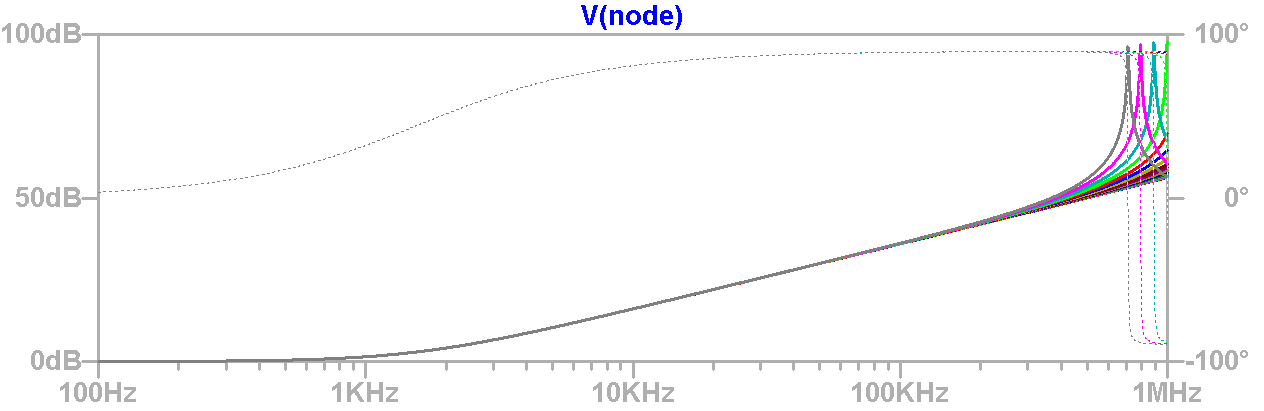# LTspice: AC Analysis Using The Step Command

In LTspice, AC analysis involves computing the AC complex node voltages as a function of frequency using an independent voltage or current source as the driving signal. The small signal analysis results are plotted in the waveform viewer as magnitude and phase over frequency.

AC analysis in LTspice has a number of settings: the X-axis scaling (linear, octave or decade), number of simulation points and frequency range. For example, if you want to see how your circuit performs from 100Hz to 1MHz with 1,000 points per decade you would edit your simulation command to the following:

.ac dec 1K 100 1Meg

Repeated AC Analysis with Parameter Sweeps

AC analysis usually involves using fixed parameters to calculate the small signal AC response of a circuit, but you may want to refine your design by viewing the response under varying parameters. This can be accomplished by stepping the parameter of interest using a .step command. For example, you could sweep a capacitance logarithmically through the range of 10pF to .5nF with 30 points per octave using the .step directive (press S to insert a spice directive in the schematic editor):

.step oct param C 10p .5n 30

The schematic for this case and its resulting waveform are shown below.Schematic of Repeated AC Analysis with Stepped Capacitance

Note that using a .step command with AC analysis can drastically increase simulation time, so carefully choose the values, ranges, increments, and frequency range for each parameter sweep.Waveform Plot of Repeated AC Analysis with Stepped Capacitance

Single Frequency Analysis with a Swept Parameter

LTspice offers an elegant solution for holding frequency constant and performing small signal analysis over a varying parameter. It is as simple as using the ‘list’ AC Analysis option in the simulation command, and specifying the frequency at which you want to perform the analysis, in this case, 1MHz:

.step oct param C 10p .5n 30
.ac list 1Meg

Using the Edit Simulation Command dialog box you can easily change the simulation from a decade to list type of sweep and only specify a single frequency.375 AC Analysis Commands Can Be Edited Using The Edit Simulation Command DialogSchematic of Single Frequency Analysis with Swept Capacitance

The beauty of single frequency analysis with a .step command is that the resulting plot shows magnitude and phase as a function of parameter sweep, not frequency. Below is the result of a simulation using a single frequency analysis where the x-axis is the capacitance sweep as defined in the .step function.Waveform Plot of Single AC Analysis with Stepped Capacitance (The x-axis is capacitance as defined by the .step command.)